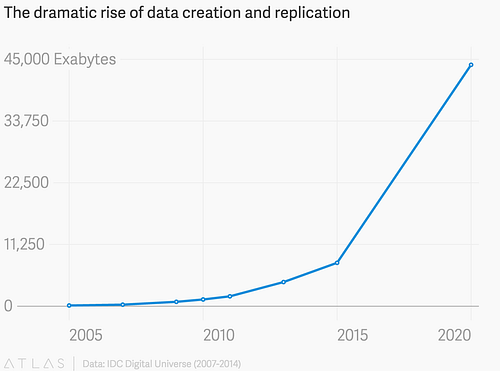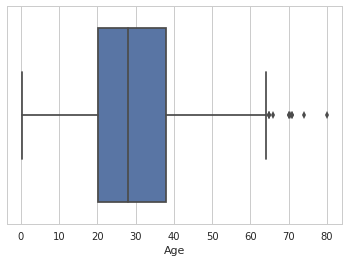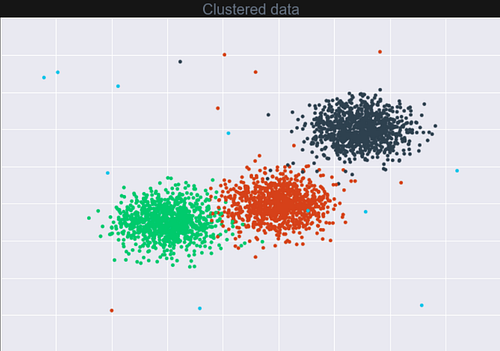## Importance of exploratory data analysis

26th November 2018With many sources predicting the exponential growth of data towards 2020 and beyond, it becomes extremely important to adopt data-intensive computing and machine learning to unlock the real value of data.This enables businesses to gain tremendous value out of the data with descriptive and predictive analytics. Most of the time problem solvers spend less time exploring data and jump straight into data modelling.

After a point of time, they struggle at improving the accuracy of their models or are just clueless about the bad performance of the model. This is because they wouldn’t have built a close relationship with the data or haven’t spent too much time understanding the data. It is not a very good idea to just feed data into a black box and wait for the results.

### Exploratory Data Analysis (EDA)

Exploratory Data Analysis is one of the important steps in the data analysis process. Before solving any Data Science problem, it is critical to have a thorough understanding of your data which can aid in sampling the right data for training, picking the right learning algorithm, etc. This can be done by spending time plotting, exploring distributions, applying general statistical methods, reviewing factor variables, etc.

The key is to formulate the correct questions to ask your data, how to manipulate the data sources to get the required answers.

### Goals of EDA

• Maximise understanding and insight into your data
• Recognise most relevant variables for the problem at hand
• Handle the outliers and anomalies present in the data
• Test hypotheses and assumptions on which statistical inferences will be based
• Obtain confidence in your data

### EDA techniques to be follow

EDA techniques mainly involve graphical visualisation methods or calculation of summary statistics. There’s high reliance on graphical methods since the main role of EDA is to open-mindedly explore, and graphics give the analysts power to do so, enable the data to reveal its structural secrets, and being always ready to gain some new, often unsuspected, insight into the data.

• Variable Identification

Identify the Predictor and Target variables (in the case of Supervised data). Once you identify these, it is crucial to analyse which type of categories these variables fall in. You can use different strategies for categorical variables and continuous variables.

• Univariate visualization of variables

Each variable needs to be analysed and the method will depend on whether the variable type is categorical or continuous.

For categorical variables, a Frequency histogram will help understand the distribution of the categories.

For continuous variables, it is best to use ‘Five-number summary’. A Five-number summary includes the median, 1st quartile, 3rd quartile minimum and maximum values of a variable. ‘Box plots’ are a graphical representation of the same. They not only visually represent five-number summary but also help find the outliers easily• Bivariate analysis of variables

The relationship between 2 variables is understood with bivariate analysis. It can be done ‘between 2 variables’ or ‘a variable and the target variable’ too. The method of analysis will depend on the combination of variables.

Categorical vs categorical variables — Stacked columned chart and Chi-Square test

Continuous vs continuous variables — Scatter plot and Correlation test

Categorical vs continuous variables — Z-Test and ANOVA

• Multivariate visualisations

Multivariate visualizations help in understanding the interactions between 3 or more variables. The usage of Contour Plots and Bubble plots are  for multivariate visualizations.

• Clustering of data points

In the case of Unsupervised data, K-means clustering works to cluster data points which aid in the understanding of the data and identify patterns of behaviour among data points. It can also be usable for finding outliers in the dataset. These points are not part of any clusters and might affect the accuracy of the model.• Outliers treatment

Outliers are those data points which have a different behaviour when compared to all other data points in the dataset. They diverge from an overall pattern in a sample. Outliers increase the error variance and also impact statistical assumptions. Various methods such as Box plots and Scatter plots can be helpful in detecting the outliers. To treat outliers, you can simply delete them  if they are less in number or transform them into the closest meaningful data point you can observe.

• Missing value treatment

Identify any missing values in your data. If a model trains with missing values, it will not learn the data as required. This can lead to wrong prediction or classification. You can use the following strategies to deal with missing values in data.

1. Delete the data points with any missing values
2. Mean/Medium/Mode imputation
3. k nearest neighbour (KNN) imputation
4. Train a predictive model which will predict the missing values based on patterns of other variables
• Variable transformation or creation

Sometimes just by changing the scale of the variable or removing skewness in the distribution of the variable; the understanding of the data to the model increases drastically. Other methods include logarithmic transformations to the variables. These are just transformations and not corrections. So, the data is interpreted differently with a transformation.

Some variables can be created out of the existing and have a better relationship with the target variable. These – now called – derived variables can be used as Predictors. An example — If there is a data point with value ‘20-Sep-1995’ in its ‘Date’ column, the creation of a ‘Year’ column and filling in only year value(1995 in this case) or creation of a ‘Day’ column with which day the date falls in (‘Wednesday’ in this case) can generate a hidden relationship.

Exploratory Data Analysis is a crucial process to perform before diving into machine learning or statistical modelling. Unfortunately, there’s not enough respect given to EDA because of impatience or ease of examples available for machine learning or deep learning implementations.

The objective of EDA is to obtain confidence in your data to a point where you’re ready to choose relevant machine learning algorithm as it provides the context needed to develop an appropriate model for the problem.

Read also: Image Inpainting with Deep Learning

Learn more about JamieAi and follow us on Twitter.I am interested in knowing the "Why" part of most things. A tech geek, an avid biker, keen thinker and passionate about finding sense in the world of data.

To give you the best possible experience, this site uses cookies. Continuing to use jamieai.com means you agree on our use of cookies.